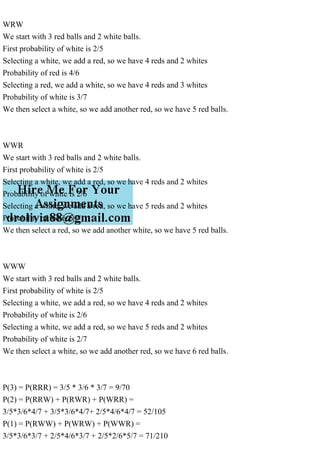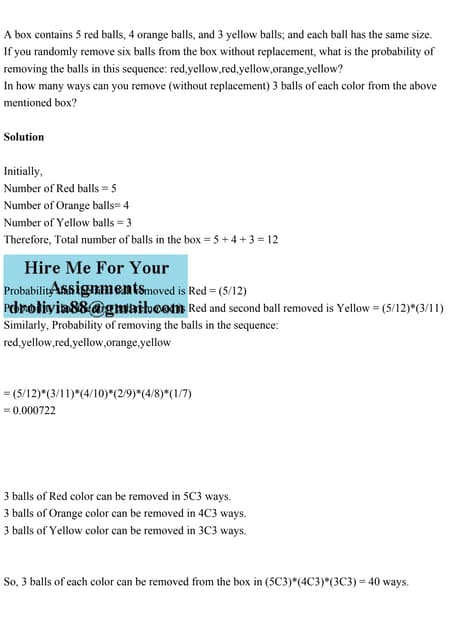PublicitéProchain SlideShareA box contains 5 red balls, 4 orange balls, and 3 yellow balls; and .pdf
Chargement dans ... 3
1 sur 4
Publicité

### An urn contains 3 red and 2 white balls. A ball is selected, replace.pdf

1. An urn contains 3 red and 2 white balls. A ball is selected, replaced, and one of the opposite color is added. This is repeated three times. Find the range distribution, mean and variance of the number X of red balls drawn. Solution The simplest way is to determine the probability of all 8 selections, then collect probabilities. RRR RRW RWR RWW WRR WRW WWR WWW RRR We start with 3 red balls and 2 white balls. First probability of red is 3/5 Selecting a red, we add a white, so we have 3 reds and 3 whites Probability of red is 3/6 Selecting a red, we add a white, so we have 3 reds and 4 whites Probability of red is 3/7 We then select a red so we add another white, so we have 3 red balls. RRW We start with 3 red balls and 2 white balls. First probability of red is 3/5